# Exercise 15.1 Introduction to Graphs- NCERT Solutions Class 8

Go back to  'Introduction to Graphs'

## Chapter 15 Ex.15.1 Question 1

The following graph shows the temperature of a patient in a hospital, recorded every hour.

(a) What was the patient’s temperature at $$1\;\rm{pm}$$?

(b) When was the patient’s temperature $$38.5^\circ\rm{C}$$?

(c) The patient’s temperature was the same two times during the period given. What were the two times?

(d) What was the temperature at $$1.30\;\rm{pm}$$? How did you arrive at your answer?

(e) During which periods did the patient’s temperature show an upward trend?

### Solution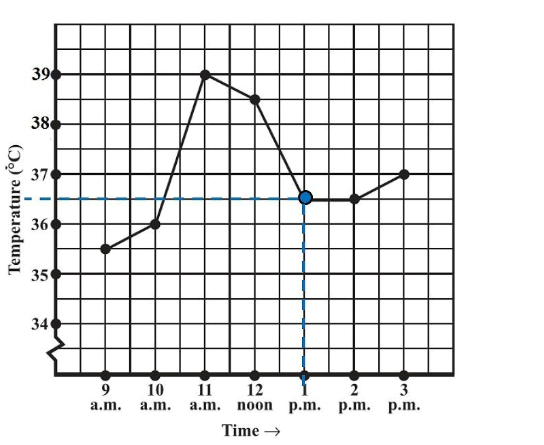Reasoning:

Horizontal axis represents Time and vertical represents Temperature in $$^\circ\,\rm{C.}$$

We can find the value of ‘Temperature’ on vertical axis according to the given value of ‘Time’ on the horizontal axis value or vice versa.

Steps:

(a) At $$1\;\rm{ pm}$$ the patient’s temperature was $$36.5^\circ\,\rm{ C.}$$

(b) The patient’s temperature was $$38.5^\circ\,\rm{ C}$$ at $$12$$ noon.

(c) The patient’s temperature was $$36.5^\circ\rm{C}$$  at $$1 \,\rm{pm}$$ and $$2 \,\rm{pm.}$$

(d) The graph between the times $$1 \,\rm{pm}$$ and $$2 \,\rm{pm}$$ is parallel to the x-axis. The temperature at $$1 \,\rm{pm}$$ and $$2 \,\rm{pm}$$ is $$36.5^\circ \;\rm{C.}$$ So, the temperature at $$1.30 \;\rm{pm}$$ is $$36.5^\circ \;\rm{C.}$$

(e) During $$9$$am to $$10 \;\rm{pm}$$, $$10 \;\rm{pm}$$ to $$11 \;\rm{pm}$$, $$2\;\rm{pm}$$  to $$3\;\rm{pm}$$ , the patient’s temperature showed an upward trend.

## Chapter 15 Ex.15.1 Question 2

The following line graph shows the yearly sales figure for a manufacturing company.

(a) What were the sales in (i) $$2002$$ (ii) $$2006$$?

(b) What were the sales in (i) $$2003$$ (ii) $$2005$$?

(c) Compute the difference between the sales in $$2002$$ and $$2006.$$

(d) In which year was there the greatest difference between the sales as compared to its previous year?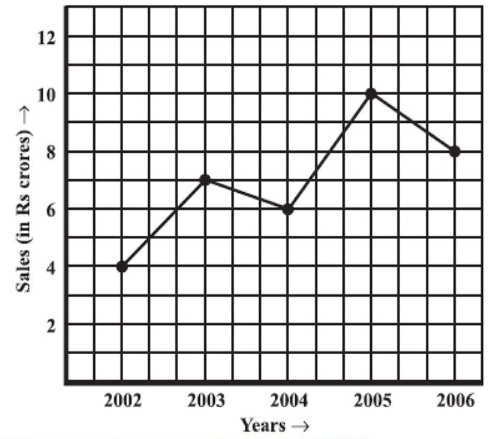### Solution

Reasoning:

Horizontal axis represents ‘Years’ and vertical represents ‘Sales’ in Rs crore.

We can find the value of ‘Sales’ on vertical axis according to the given value of ‘Years’ on the horizontal axis value or vice versa.

Steps:

(a)

(i) In $$2002,$$ the sales were $$\rm{Rs}\; 4$$ crores.

(ii) In $$2006,$$ the sales were $$\rm{Rs}\; 8$$ crores.

(b)

(i) In $$2003,$$ the sales were $$\rm{Rs}\; 7$$ crores.

(ii) In $$2005,$$ the sales were $$\rm{Rs}\; 10$$ crores.

(c)

(i) In $$2002,$$ the sales were $$\rm{Rs}\; 4$$ crores and in $$2006,$$ the sales were $$\rm{Rs}\; 8$$ crores.

Difference between the sales in $$2002$$ and $$2006$$

$$= \rm{Rs} \;(8 − 4)$$ crores $$=$$ $$\rm{Rs}\; 4$$ crores

(d) Difference between the sales of the year $$2006$$ and $$2005$$

$$= \rm{Rs}\; (10 − 8)$$ crores $$=\rm{ Rs}\; 2$$ crores

Difference between the sales of the year $$2005$$ and $$2004$$

$$= \rm{Rs}\; (10 − 6)$$ crores $$=\rm{Rs}\; 4$$ crores

Difference between the sales of the year $$2004$$ and $$2003$$

$$=\rm{Rs}\; (7 − 6)$$ crore $$= \rm{Rs}\; 1$$ crore

Difference between the sales of the year $$2003$$ and $$2002$$

$$= \rm{Rs} \;(7 − 4)$$ crores $$=\rm{Rs}\; 3$$ crores

Hence, the difference was the maximum in the year $$2005$$ as compared to its previous year $$2004.$$

## Chapter 15 Ex.15.1 Question 3

For an experiment in Botany, two different plants, plant $$A$$ and plant $$B$$ were grown under similar laboratory conditions. Their heights were measured at the end of each week for $$3$$ weeks. The results are shown by the following graph.

(a) How high was Plant $$A$$ after (i) $$2$$ weeks (ii) $$3$$ weeks?

(b) How high was Plant $$B$$ after (i) $$2$$ weeks (ii) $$3$$ weeks?

(c) How much did Plant $$A$$ grow during the $$3^\rm{rd}$$ week?

(d) How much did Plant $$B$$ grow from the end of the $$2^\rm{nd}$$ week to the end of the $$3^\rm{rd}$$ week?

(e) During which week did Plant $$A$$ grow most?

(f) During which week did Plant $$B$$ grow least?

(g) Were the two plants of the same height during any week shown here? Specify.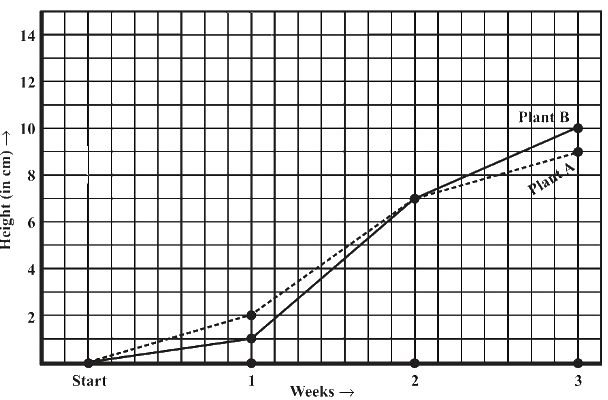### Solution

Reasoning:

Horizontal axis represents ‘Weeks’ and vertical represents ‘Height’ in $$\rm{cm.}$$

We can find the value of ‘Height’ on vertical axis according to the given value of ‘Weeks’ on the horizontal axis value or vice versa.

Steps:

(a) How high was Plant $$A$$ after (i) $$2$$ weeks (ii) $$3$$ weeks?

(i) After $$2$$ weeks, the height of plant $$A$$ was $$7 \;\rm{cm.}$$

(ii) After $$3$$ weeks, the height of plant A was $$9 \;\rm{cm.}$$

(b) How high was Plant $$B$$ after (i) $$2$$ weeks (ii) $$3$$ weeks?

(i) After $$2$$ weeks, the height of plant $$B$$ was $$7 \;\rm{cm.}$$

(ii) After $$3$$ weeks, the height of plant $$B$$ was $$10 \;\rm{cm.}$$

(c) Growth of plant $$A$$ during $$3$$rd week $$= 9\;\rm{cm} − 7\;\rm{cm} = 2\;\rm{cm}$$

(d) Growth of plant $$B$$ from the end of the $$2^\rm{nd}$$ week to the end of the $$3 ^\rm{rd}$$ week

$$= 10\, \rm{cm} − 7 \, \rm{cm} = 3 \, \rm{cm}$$

(e) Growth of plant $$A$$ during $$1^\rm{st}$$ week $$= 2\,\rm{cm } − 0 \,\rm{cm } = 2 \,\rm{cm }$$

Growth of plant $$A$$ during $$2^\rm{nd}$$week $$= 7 \,\rm{cm} − 2 \,\rm{cm} = 5 \,\rm{cm}$$

Growth of plant A during $$3^\rm{rd}$$ week $$= 9\,\rm{cm} − 7 \,\rm{cm} = 2 \,\rm{cm}$$

Therefore, plant $$A$$ grew the most, i.e. $$5 \,\rm{cm,}$$ during the $$2^\rm{nd}$$ week.

(f) Growth of plant $$B$$ during $$1^\rm{st}$$ week $$= 1\,\rm{cm} − 0 \,\rm{cm} = 1 \,\rm{cm}$$

Growth of plant $$B$$ during $$2^\rm{nd}$$ week $$= 7\,\rm{cm} − 1\,\rm{cm} = 6 \,\rm{cm}$$

Growth of plant $$B$$ during $$3^\rm{rd}$$ week $$= 10 \,\rm{cm} − 7\,\rm{cm} = 3\,\rm{cm}$$

Therefore, plant $$B$$ grew the least, i.e. $$1 \;\rm{cm,}$$ during the $$1^\rm{st}$$ week.

(g) At the end of the $$2^\rm{nd}$$ week, the heights of both plants were same .i.e. $$7 \rm{cm}$$.

## Chapter 15 Ex.15.1 Question 4

The following graph shows the temperature forecast and the actual temperature for each day of a week.

(a) On which days was the forecast temperature the same as the actual temperature?

(b) What was the maximum forecast temperature during the week?

(c) What was the minimum actual temperature during the week?

(d) On which day did the actual temperature differ the most from the forecast temperature?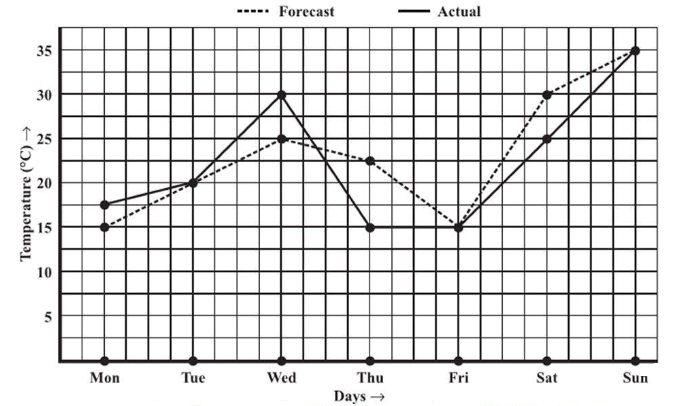### Solution

Reasoning:

Horizontal axis represents ‘Days’ and vertical represents ‘Temperature’ in $$\rm{C\;^\circ}$$

We can find the value of ‘Temperature’ on vertical axis according to the given value of ‘Days’ on the horizontal axis value or vice versa.

Steps:

(a) The forecast temperature was same as the actual temperature on Tuesday, Friday, and Sunday.

(b) The maximum forecast temperature during the week was $$35^\circ \rm{C.}$$

(c) The minimum actual temperature during the week was $$15^\circ\rm{C.}$$

(d) The actual temperature differs the most from the forecast temperature on Thursday.

## Chapter 15 Ex.15.1 Question 5

Use the tables below to draw linear graphs.

(a) The number of days a hill side city received snow in different years.

 Year $$2003$$ $$2004$$ $$2005$$ $$2006$$ Days $$8$$ $$10$$ $$5$$ $$12$$

(b) Population (in thousands) of men and women in a village in different years.

 Year $$2003$$ $$2004$$ $$2005$$ $$2006$$ $$2007$$ Number of men $$12$$ $$12.5$$ $$13$$ $$13.2$$ $$13.5$$ Number of Women $$11.3$$ $$11.9$$ $$13$$ $$13.6$$ $$12.8$$

### Solution

Reasoning:

(a) Considering ‘Years’ on horizontal axis and ‘Days’ on vertical axis starting from origin i.e. ($$0,0$$).

(b) Considering ‘Years’ on horizontal axis and ‘Number of persons (i.e. ‘Men’ or ‘Women’) on vertical axis starting from origin i.e. ($$0,0$$). Represent ‘Number of men’ as a straight continuous line where as ‘Number of Women’ as a discontinuous straight line ( dashed line).

Steps:

(a) By taking the years on $$x$$-axis and the number of days on $$y$$-axis and taking scale as $$1$$ unit $$= 2$$ days on $$y$$-axis and $$2$$ unit $$= 1$$ year on $$x$$-axis, the linear graph of the given information can be drawn as follows.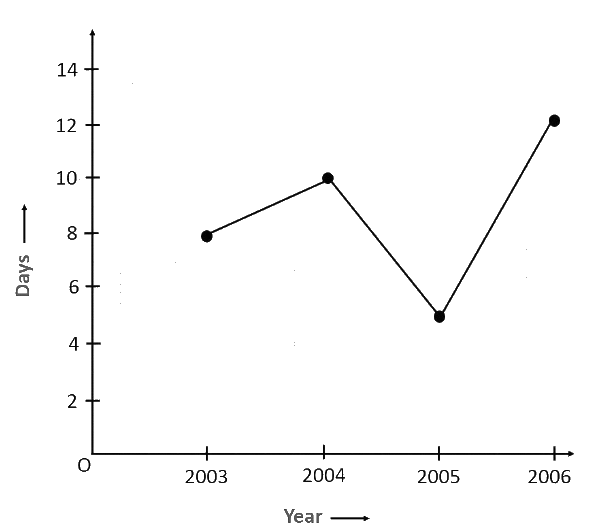(b) By taking the years on $$x$$-axis and population on $$y$$-axis and scale as $$1$$ unit $$= 0.5$$ thousand on $$y$$-axis and $$2$$ unit $$=$$$$1$$ year on $$x$$-axis, the linear graph of the given information can be drawn as follows.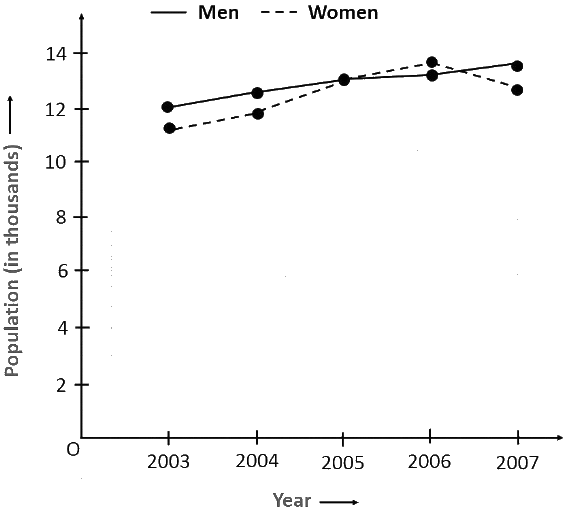## Chapter 15 Ex.15.1 Question 6

A courier-person cycles from a town to a neighboring suburban area to deliver a parcel to a merchant. His distance from the town at different times is shown by the following graph.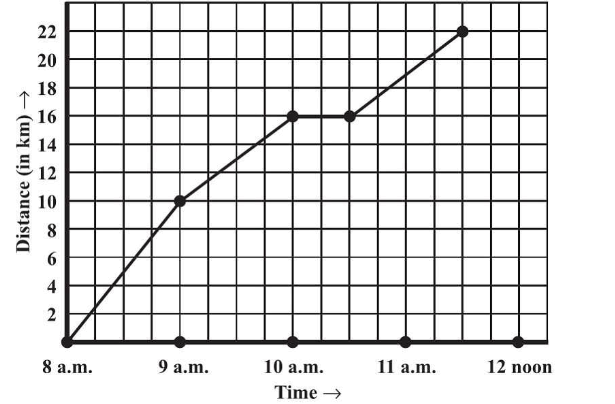(a) What is the scale taken for the time axis?

(b) How much time did the person take for the travel?

(c) How far is the place of the merchant from the town?

(d) Did the person stop on his way? Explain.

(e) During which period did he ride fastest?

### Solution

Reasoning:

Horizontal axis represents ‘Time’ and vertical represents ‘Distance’ in $$\rm{km.}$$

We can find the value of ‘Distance’ on vertical axis according to the given value of ‘Time’ on the horizontal axis value or vice versa.

Steps:

(a) Scale taken for the time axis  i.e. x -axis is $$4$$ units $$= 1$$ hour

(b) The person travelled during the time $$8 \;\rm{a.m.} − 11:30 \;\rm{a.m.}$$

Therefore, the person took \begin{align}3\frac{1}{2}\end{align} hours to travel.

(c) The place of the merchant is $$22\,\rm{ km}$$ far from the town.

(d) Yes, the person stopped on his way from $$10\,\rm{ a.m.}$$ to $$10: 30\;\rm{a.m.}$$ This is indicated by the horizontal part of the graph.

(e) From the graph, it can be observed that during $$8 \;\rm{a.m.}$$ to $$9\;\rm{ a.m.},$$ the person travelled the maximum distance. Thus, the person’s ride was the fastest between $$8 \;\rm{a.m.}$$ and $$9 \;\rm{a.m.}$$

## Chapter 15 Ex.15.1 Question 7

Can there be a time temperature graph as follows? Justify you’re answer: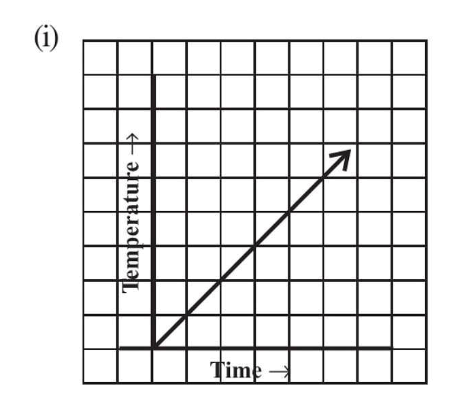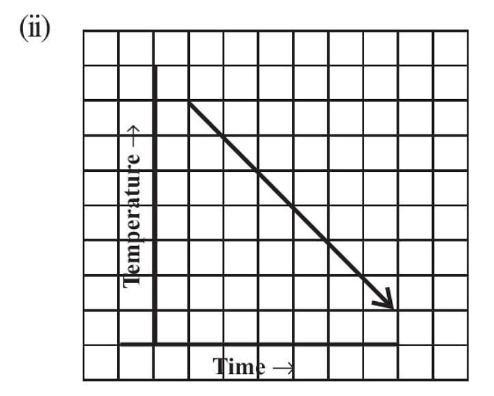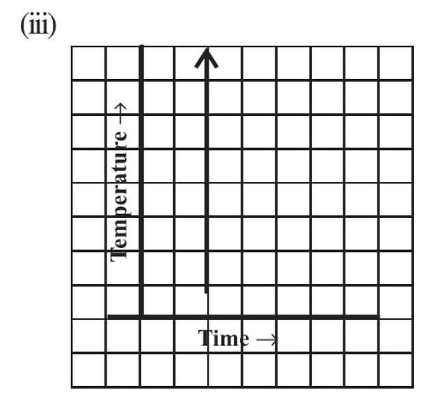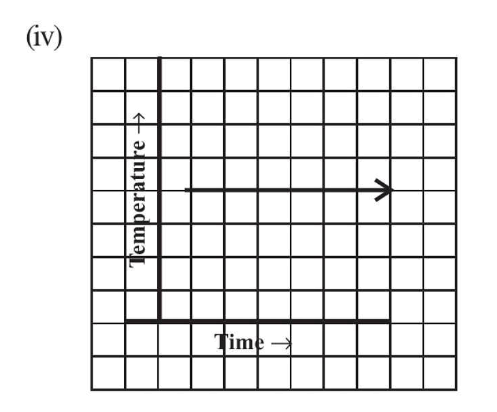### Solution

Reasoning:

We cannot have different temperatures at the same time.

Steps:

(i) This can be a time−temperature graph, as the temperature can increase with the increase in time.

(ii) This can be a time−temperature graph, as the temperature can decrease with the decrease in time.

(iii) This cannot be a time−temperature graph since different temperatures at the same time are not possible.

(iv) This can be a time−temperature graph, as same temperature at different times is possible.

Related Sections
Related Sections
Instant doubt clearing with Cuemath Advanced Math Program